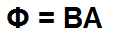﻿ Magnetic Flux Calculator ﻿# Magnetic Flux Calculator

### Magnetic Flux Calculator If Magnetic Field, B, is at a 90° angle (Perpendicular) to Area, AMagnetic Field, B: tesla Area, A: m2
0.00 webers

This magnetic flux calculator calculates the magnetic flux of an object based on the magnitude of the magnetic field which the object emanates and the area of the object, according to the formula, Φ=BA, if the magnetic field is at a 90° angle (perpendicular) to the area of the object. If the magnetic field is not perpendicular to the object, then use the calculator below, which computes the magnetic flux at non-perpendicular angles.

To use this calculator, a user just enters the magnetic field, B, and the area, A, of the object, and clicks the 'Submit' button. The resultant value will automatically be computed and shown below. The unit which the magnetic field is computed in is unit tesla. The unit which the area is computed in is in unit meters squared (m2). The resultant value of the magnetic flux is webers.

The magnetic flux is directly proportional to the magnitude of the magnetic field emanating from the object and the area of the object. The greater the magnetic field, the greater the magnetic flux. Conversely, the smaller the magnetic field, the smaller the flux. The area of the object has the same direct relationship. The greater the area of an object, the greater the flux. Conversely, the smaller the area, the smaller the magnetic flux.

### Magnetic Flux Calculator If Magnetic Field, B, is at a non-90° angle (non-perpendicular) to Area, AMagnetic Field, B: tesla Area, A: m2 Angle, α: ° rad
0.00 webers

This magnetic flux calculator calculates the magnetic flux of an object based on the magnitude of the magnetic field which the object emanates, the area of the object, and the angle of the object from the 90° perpendicular angle, according to the formula, Φ= BAcos(α), if the magnetic field is at a non-90° angle (non-perpendicular) to the area of the object. If the magnetic field isperpendicular to the object, then use the calculator above, which computes the magnetic flux at perpendicular angles.

To use this calculator, a user just enters the magnetic field, B, the area, A, and the angle, α, of the object, and clicks the 'Submit' button. The resultant value will automatically be computed and shown below. The unit which the magnetic field is computed in is unit tesla. The unit which the area is computed in is in unit meters squared (m2). The unit of the angle, α, can be either in radians or degrees. The resultant value of the magnetic flux is in unit webers.

The magnetic flux, again, has a direct relationship with the magnitude of the magnetic field and the area of the object. The greater the magnetic field or area, the greater the flux. The smaller the field or the area, the smaller the flux.

Magnetic flux is an important calculation in engineering and in circuits, because some circuit components store magnetic fields as energy. The best example of this is an inductor. An inductor is a device which creates a magnetic field when currents run through it. Being that it creates a magnetic field, it has magnetic flux. This magnetic flux can then penetrate a nearby object and cause either intended or unintended effects for a circuit. Being able to calculate the magnetic flux to gauge its strength, a designer can better understand quantitatively what this flux can do to a circuit.

Related Resources

﻿